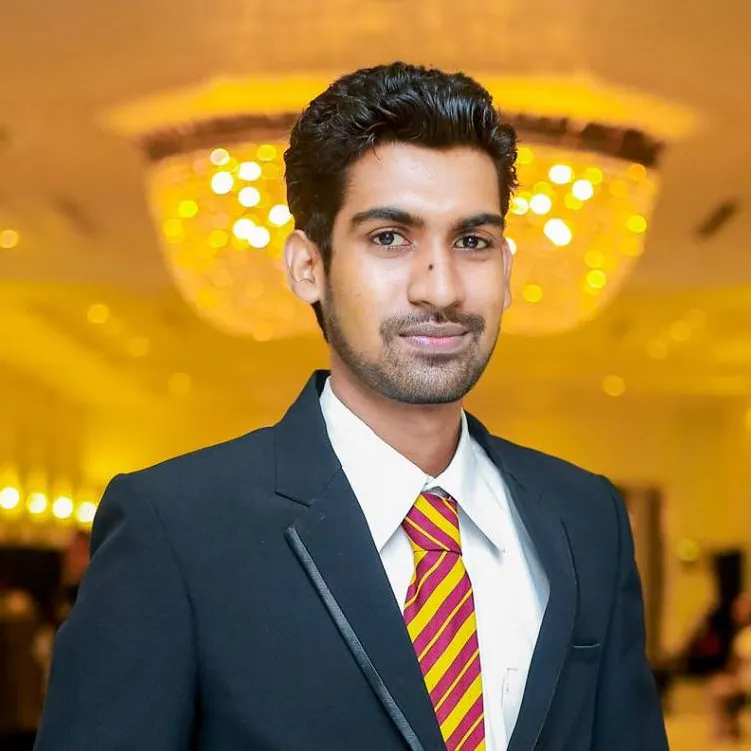# Portable Environment Monitor

A handheld monitor for young scientists to get familiar with various aspects of the surrounding environment.

IntermediateFull instructions provided5 hours3,736

## Things used in this project

### Hardware components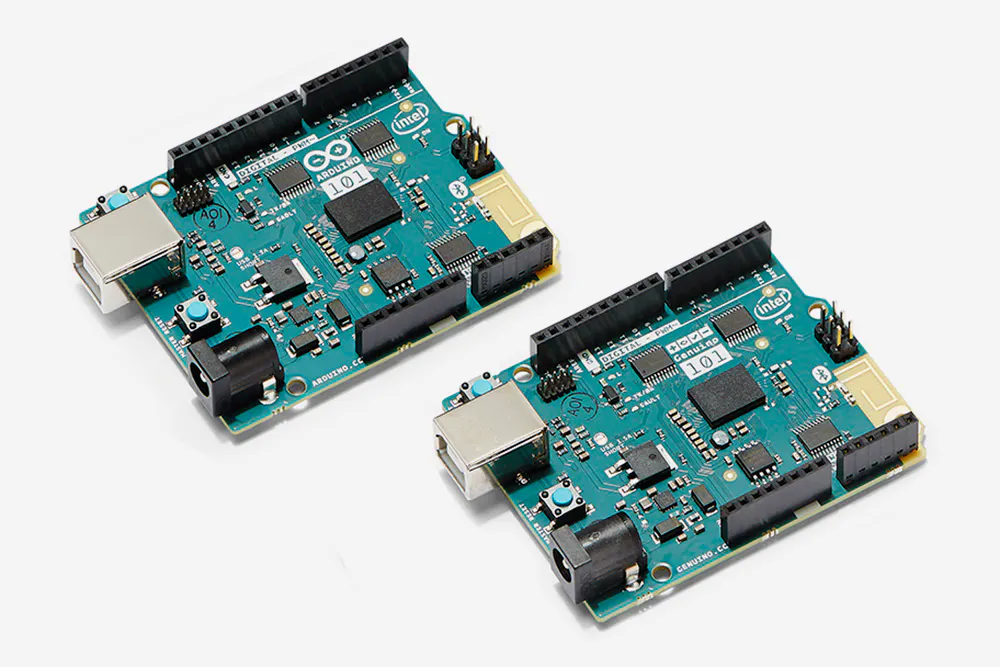Arduino 101 & Genuino 101
×1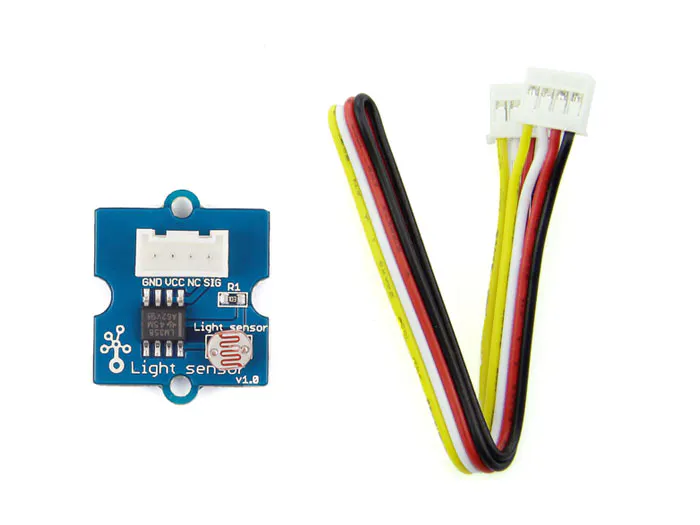Seeed Grove - Light Sensor
×1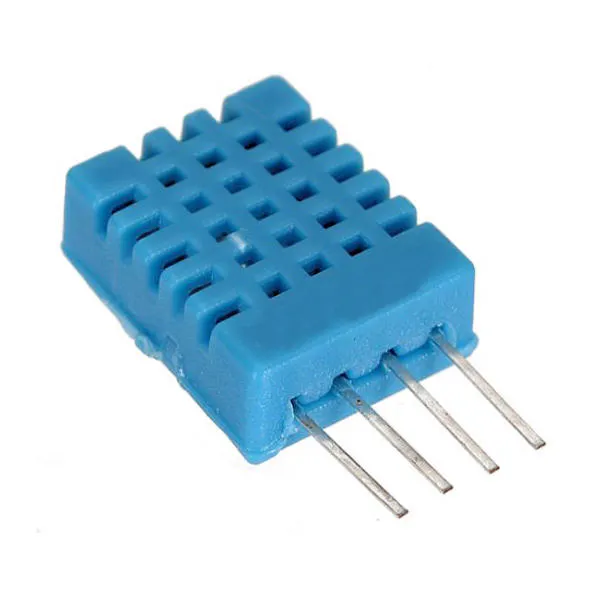DHT11 Temperature & Humidity Sensor (4 pins)
×1
 Sparkfun Sound Detector
×1
 Seeed Grove LCD
×1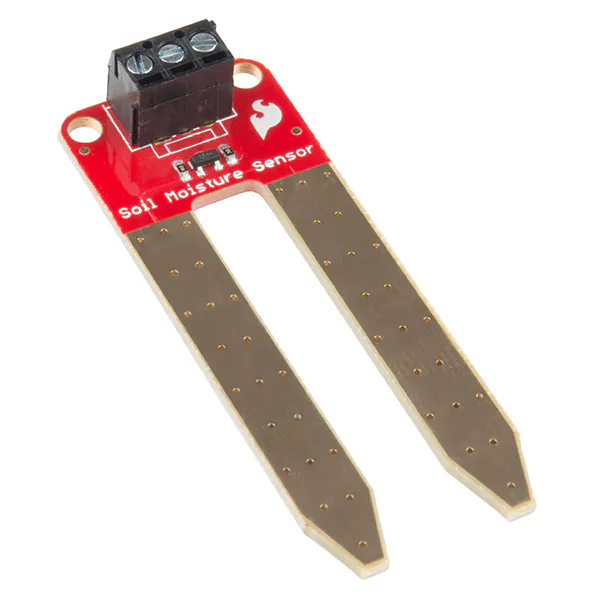SparkFun Soil Moisture Sensor (with Screw Terminals)
×1
 Seeed Grove Button
×1
 Seeed Grove universal cables
×1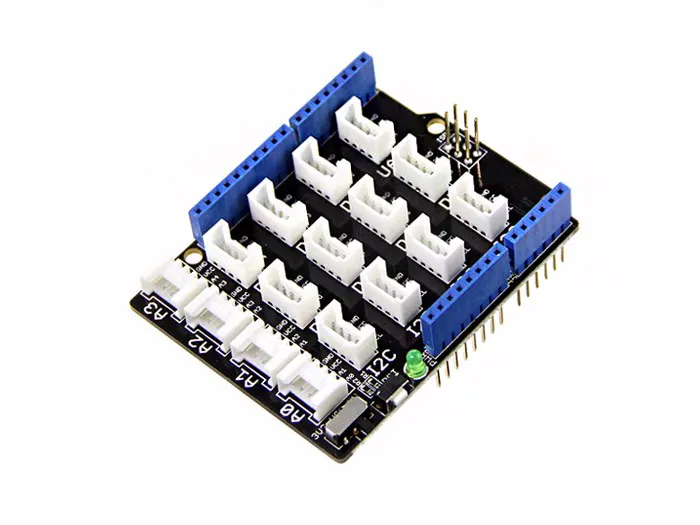Seeed Base Shield V2
×1

### Software apps and online servicesArduino IDE

## Code

### Basic_1.ino

Arduino
``` #include <Adafruit_Sensor.h>
#include "DHT.h"         //include DHT library
#define DHTPIN 3         //define as DHTPIN the Pin 3 used to connect the Sensor
#define DHTTYPE DHT11    //define the sensor used(DHT11)
#define light A0
#define noise A1
#define moist A2
#define button 8
DHT dht(DHTPIN, DHTTYPE);//create an instance of DHT
#include <Wire.h>
#include "rgb_lcd.h"
#include "math.h"

rgb_lcd lcd;

const int colorR = 0;
const int colorG = 30;
const int colorB = 55;

int page = 0;

void setup()
{
// set up the LCD's number of columns and rows:
Serial.begin(9600);
lcd.begin(16, 2);
dht.begin();
lcd.setRGB(colorR, colorG, colorB);
pinMode(light,INPUT);
pinMode(noise,INPUT);
pinMode(button,INPUT);
pinMode (2,OUTPUT);
pinMode (A3,OUTPUT);
digitalWrite(2,HIGH);
digitalWrite(A3,OUTPUT);
pinMode (1,OUTPUT);
}

void loop()
{
while (page == 0){
first_page();
page++;
}
delay(500);
while (page == 1){
second_page();
page++;
}
delay(500);

while (page == 2){
third_page();
page = 0;
}

delay(500);

}

void first_page(){

int light_l = 0;

for (int x=0; x<50;x++){
delay(2);
}

light_l = light_l/50;
lcd.setCursor(12, 1);
lcd.print("  lx");
lcd.setCursor(0, 0);
lcd.print("Temp ");
lcd.setCursor(0, 1);
lcd.print(t);
lcd.setCursor(2, 1);
lcd.print("_C ");

lcd.setCursor(5, 0);
lcd.print("Humid");
lcd.setCursor(6, 1);
lcd.print(h);
lcd.setCursor(8, 1);
lcd.print("%");

lcd.setCursor(11, 0);
lcd.print("Light");
lcd.setCursor(11, 1);
lcd.print(light_l);

}
void second_page(){

int sound = 0;
for(int x = 0;x<10;x++){
}
sound = sound/10;
double dB = 20*(log10(10*sound)) ;
lcd.setCursor(0, 0);
lcd.print("Noise           ");
lcd.setCursor(0, 1);
lcd.print(dB);
lcd.setCursor(5, 1);
lcd.print("            ");
delay(100);

}

void third_page(){
int moist_s = 0;

for (int y = 0; y<100 ; y++){
delay(2);
}

moist_s = moist_s/100;
lcd.setCursor(0,0);
lcd.print("Water- ");
lcd.setCursor(0,1);
lcd.print("Soil- ");

lcd.setCursor(6,1);
if(moist_s>900) lcd.print("Dry    ");
else if(moist_s>650) lcd.print("Medium");
else lcd.print("High   ");

lcd.setCursor(7,0);
if(moist_s>750) lcd.print("No      ");
else if(moist_s>600) lcd.print("Distill ");
else if(moist_s>500) lcd.print("Drinking");
else if(moist_s>350) lcd.print("Fish    ");
else lcd.print("Polluted");

}
```

### DHT11.ino

Arduino
```// DHT11 Temperature and Humidity Sensors Example
#include "DHT.h"         //include DHT library
#define DHTPIN 3         //define as DHTPIN the Pin 3 used to connect the Sensor
#define DHTTYPE DHT11    //define the sensor used(DHT11)
DHT dht(DHTPIN, DHTTYPE);//create an instance of DHT
/*setup*/
void setup() {
Serial.begin(9600);    //initialize the Serial communication
delay(6000);           //wait 6 seconds
Serial.println("Temperature and Humidity test!");//print on Serial monitor
Serial.println("T(C) \tH(%)");                   //print on Serial monitor
dht.begin();           //initialize the Serial communication
}
/*loop*/
void loop() {

// check if any reads failed and exit early (to try again).
if (isnan(h) || isnan(t)) {
Serial.println("Failed to read from DHT sensor!");
return;
}
Serial.print(t, 2);    //print the temperature
Serial.print("\t");
Serial.println(h, 2);  //print the humidity
delay(500);           //wait 2 seconds
}
```

### Kalman.h

C/C++
```/* Copyright (C) 2012 Kristian Lauszus, TKJ Electronics. All rights reserved.

This software may be distributed and modified under the terms of the GNU
Foundation and appearing in the file GPL2.TXT included in the packaging of
this file. Please note that GPL2 Section 2[b] requires that all works based
on this software must also be made publicly available under the terms of
the GPL2 ("Copyleft").

Contact information
-------------------

Kristian Lauszus, TKJ Electronics
Web      :  http://www.tkjelectronics.com
e-mail   :  kristianl@tkjelectronics.com
*/

#ifndef _Kalman_h
#define _Kalman_h

class Kalman {
public:
Kalman() {
/* We will set the variables like so, these can also be tuned by the user */
Q_angle = 0.01;//0.001
Q_bias = 0.0001;//0.003
R_measure = 0.11;//0.03

angle = 0; // Reset the angle
bias = 0; // Reset bias

P = 0; // Since we assume that the bias is 0 and we know the starting angle (use setAngle), the error covariance matrix is set like so - see: http://en.wikipedia.org/wiki/Kalman_filter#Example_application.2C_technical
P = 0;
P = 0;
P = 0;
};
// The angle should be in degrees and the rate should be in degrees per second and the delta time in seconds
double getAngle(double newAngle, double newRate, double dt) {
// KasBot V2  -  Kalman filter module - http://www.x-firm.com/?page_id=145
// Modified by Kristian Lauszus

// Discrete Kalman filter time update equations - Time Update ("Predict")
// Update xhat - Project the state ahead
/* Step 1 */
rate = newRate - bias;
angle += dt * rate;

// Update estimation error covariance - Project the error covariance ahead
/* Step 2 */
P += dt * (dt*P - P - P + Q_angle);
P -= dt * P;
P -= dt * P;
P += Q_bias * dt;

// Discrete Kalman filter measurement update equations - Measurement Update ("Correct")
// Calculate Kalman gain - Compute the Kalman gain
/* Step 4 */
S = P + R_measure;
/* Step 5 */
K = P / S;
K = P / S;

// Calculate angle and bias - Update estimate with measurement zk (newAngle)
/* Step 3 */
y = newAngle - angle;
/* Step 6 */
angle += K * y;
bias += K * y;

// Calculate estimation error covariance - Update the error covariance
/* Step 7 */
P -= K * P;
P -= K * P;
P -= K * P;
P -= K * P;

return angle;
};
void setAngle(double newAngle) { angle = newAngle; }; // Used to set angle, this should be set as the starting angle
double getRate() { return rate; }; // Return the unbiased rate

/* These are used to tune the Kalman filter */
void setQangle(double newQ_angle) { Q_angle = newQ_angle; };
void setQbias(double newQ_bias) { Q_bias = newQ_bias; };
void setRmeasure(double newR_measure) { R_measure = newR_measure; };

double getQangle() { return Q_angle; };
double getQbias() { return Q_bias; };
double getRmeasure() { return R_measure; };

private:
/* Kalman filter variables */
double Q_angle; // Process noise variance for the accelerometer
double Q_bias; // Process noise variance for the gyro bias
double R_measure; // Measurement noise variance - this is actually the variance of the measurement noise

double angle; // The angle calculated by the Kalman filter - part of the 2x1 state vector
double bias; // The gyro bias calculated by the Kalman filter - part of the 2x1 state vector
double rate; // Unbiased rate calculated from the rate and the calculated bias - you have to call getAngle to update the rate

double P; // Error covariance matrix - This is a 2x2 matrix
double K; // Kalman gain - This is a 2x1 vector
double y; // Angle difference
double S; // Estimate error
};

#endif
```

### Final Code

Arduino
```#include <Adafruit_Sensor.h>
#include "DHT.h"         //include DHT library
#define DHTPIN 3         //define as DHTPIN the Pin 3 used to connect the Sensor
#define DHTTYPE DHT11    //define the sensor used(DHT11)
#define light A0
#define noise A1
#define moist A2
#define button 8
DHT dht(DHTPIN, DHTTYPE);//create an instance of DHT
#include <Wire.h>
#include "rgb_lcd.h"
#include "math.h"
#include <CurieIMU.h>
#include "Kalman.h"

Kalman kalmanX; // Create the Kalman instances
Kalman kalmanY;

float accX, accY, accZ;
float gyroX, gyroY, gyroZ;

double gyroXangle, gyroYangle;
double compAngleX, compAngleY;
double kalAngleX, kalAngleY;

rgb_lcd lcd;
uint32_t timer;

const int colorR = 0;
const int colorG = 30;
const int colorB = 55;

int page = 0;

void setup()
{
// set up the LCD's number of columns and rows:
Serial.begin(9600);
lcd.begin(16, 2);
dht.begin();
lcd.setRGB(colorR, colorG, colorB);
pinMode(light,INPUT);
pinMode(noise,INPUT);
pinMode(button,INPUT);
pinMode (2,OUTPUT);
pinMode (A3,OUTPUT);
digitalWrite(2,HIGH);
digitalWrite(A3,OUTPUT);
pinMode (1,OUTPUT);
CurieIMU.begin();
CurieIMU.setAccelerometerRange(2);
CurieIMU.setGyroRange(250);
delay(100); // Wait for sensor to stabilize

double roll  = atan2(accY,accZ) * RAD_TO_DEG;
double pitch = atan(-accX / sqrt(accY * accY + accZ * accZ)) * RAD_TO_DEG;
//double yaw = atan (accZ/sqrt(accX*accX + accZ*accZ)) * RAD_TO_DEG;
kalmanX.setAngle(roll); // Set starting angle
kalmanY.setAngle(pitch);
gyroXangle = roll;
gyroYangle = pitch;
}

void loop()
{
while (page == 0){
first_page();
page++;
}
delay(500);
while (page == 1){
second_page();
page++;
}
delay(500);

while (page == 2){
third_page();
page = 0;
}

delay(500);

}

void first_page(){

int light_l = 0;

for (int x=0; x<50;x++){
delay(2);
}

light_l = light_l/50;
lcd.setCursor(12, 1);
lcd.print("  lx");
lcd.setCursor(0, 0);
lcd.print("Temp ");
lcd.setCursor(0, 1);
lcd.print(t);
lcd.setCursor(2, 1);
lcd.print("_C ");

lcd.setCursor(5, 0);
lcd.print("Humid");
lcd.setCursor(6, 1);
lcd.print(h);
lcd.setCursor(8, 1);
lcd.print("%");

lcd.setCursor(11, 0);
lcd.print("Light");
lcd.setCursor(11, 1);
lcd.print(light_l);

}
void second_page(){

int sound = 0;
for(int x = 0;x<10;x++){
}
sound = sound/10;
double dB = 20*(log10(10*sound)) ;
lcd.setCursor(0, 0);
lcd.print("Noise ");
lcd.setCursor(0, 1);
lcd.print(dB,0);
lcd.setCursor(2, 1);
lcd.print("dB");
lcd.setCursor(6, 0);
lcd.print("Roll ");
lcd.setCursor(11, 0);
lcd.print("Pitch");

for (int z=0; z<10;z++){

double dt = (double)(micros() - timer) / 1000000; // Calculate delta time
timer = micros();

double roll  = atan2(accY,accZ) * RAD_TO_DEG;
double pitch = atan(-accX / sqrt(accY * accY + accZ * accZ)) * RAD_TO_DEG;
//double yaw = atan2(accZ,sqrt(accX*accX + accZ*accZ)) * RAD_TO_DEG;

double gyroXrate = gyroX / 131.0; // Convert to deg/s
double gyroYrate = gyroY / 131.0; // Convert to deg/s

if ((roll < -90 && kalAngleX > 90) || (roll > 90 && kalAngleX < -90)) {
kalmanX.setAngle(roll);
compAngleX = roll;
kalAngleX = roll;
gyroXangle = roll;
} else
kalAngleX = kalmanX.getAngle(roll, gyroXrate, dt); // Calculate the angle using a Kalman filter

if (abs(kalAngleX) > 90)
gyroYrate = -gyroYrate; // Invert rate, so it fits the restriced accelerometer reading
kalAngleY = kalmanY.getAngle(pitch, gyroYrate, dt);

gyroXangle += gyroXrate * dt; // Calculate gyro angle without any filter
gyroYangle += gyroYrate * dt;
//gyroXangle += kalmanX.getRate() * dt; // Calculate gyro angle using the unbiased rate
//gyroYangle += kalmanY.getRate() * dt;

compAngleX = 0.93 * (compAngleX + gyroXrate * dt) + 0.07 * roll; // Calculate the angle using a Complimentary filter
compAngleY = 0.93 * (compAngleY + gyroYrate * dt) + 0.07 * pitch;

if (gyroXangle < -180 || gyroXangle > 180)
gyroXangle = kalAngleX;
if (gyroYangle < -180 || gyroYangle > 180)
gyroYangle = kalAngleY;

delay(10);
}

lcd.setCursor(10,1);
lcd.print("  ");
lcd.setCursor(6,1);
lcd.print(kalAngleX,1);
lcd.setCursor(15,1);
lcd.print(" ");
lcd.setCursor(12,1);
lcd.print(kalAngleY,1);

}

void third_page(){
int moist_s = 0;

for (int y = 0; y<100 ; y++){
delay(2);
}

moist_s = moist_s/100;
lcd.setCursor(0,0);
lcd.print("Water- ");
lcd.setCursor(0,1);
lcd.print("Soil- ");

lcd.setCursor(6,1);
if(moist_s>900) lcd.print("Dry       ");
else if(moist_s>650) lcd.print("Medium");
else lcd.print("High   ");

lcd.setCursor(7,0);
if(moist_s>750) lcd.print("No       ");
else if(moist_s>600) lcd.print("Distill ");
else if(moist_s>500) lcd.print("Drinking");
else if(moist_s>350) lcd.print("Fish    ");
else lcd.print("Polluted");

}
```

## Credits

### Chathuranga Liyanage

1 project • 24 followers
Electronic Engineer Specialized in Mobile Robotics with more than 5 years of experience